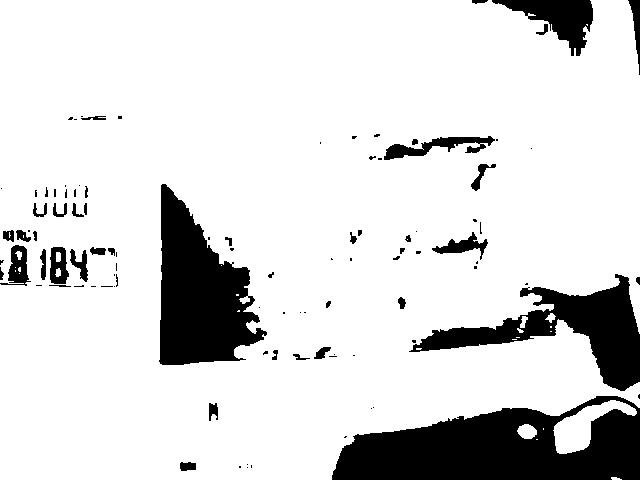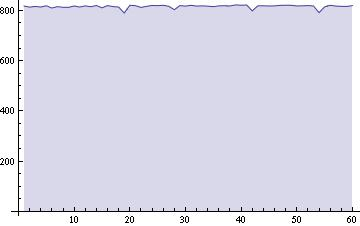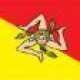# OCR’ing a power meter

The following shows the output of the IP camera which is photographing the power meter (what the photo doesn’t show is a large high powered light just above the power meter, to keep the output nice and clear):We then threshold the image so that the numbers are clearly recognizable:Seven points where then taken from the LCD segments and mapped to a list, we are then able to compare the list to known values for different digits:We moved the points across the image to detect each of the digits on the power meter.

The following shows the output from 1 hours worth of samples from a blade server, taken each minute:The following is the Mathematica sourcecode:

```datav = {}

For][];

powermeter =
Binarize[GaussianFilter[Binarize[powermeter, 0.27], 1], 0.20];

output = 0;
For[z = 0, z <= 3, z = z + 1,
x = z*20;
seg = {ImageValue[powermeter, {73 - x, 225}],
ImageValue[powermeter, {79 - x, 231}],
ImageValue[powermeter, {84 - x, 220}],
ImageValue[powermeter, {78 - x, 216}],
ImageValue[powermeter, {72 - x, 209}],
ImageValue[powermeter, {78 - x, 201}],
ImageValue[powermeter, {84 - x, 206}]};

digit = -1;
If[seg == {0, 0, 0, 1, 0, 0, 0}, digit = 0] ;
If[seg == {1, 1, 0, 1, 1, 1, 0}, digit = 1];
If[seg == {1, 0, 0, 0, 0, 0, 1}, digit = 2];
If[seg == {1, 0, 0, 0, 1, 0, 0}, digit = 3];
If[seg == {0, 1, 0, 0, 1, 1, 0}, digit = 4];
If[seg == {0, 0, 1, 0, 1, 0, 0}, digit = 5];
If[seg == {0, 0, 1, 0, 0, 0, 0}, digit = 6];
If[seg == {1, 0, 0, 1, 1, 1, 0}, digit = 7];
If[seg == {0, 0, 0, 0, 0, 0, 0}, digit = 8];
If[seg == {0, 0, 0, 0, 1, 0, 0}, digit = 9];
output += digit*(10^(z - 1));

];

If[output <= 300, output *= 10];
datav  = Append[datav, output];
Pause;
]

ListLinePlot[datav, {Filling -> Axis, AxesOrigin -> {0, 0}}]

```

As it’s hard to recognize the decimal point in the image, I use knowledge of the fact my blade server is unlikely to be running at <=300W so it should in-fact be running at 3000W. This is accounted for in this line: If[output <= 300, output *= 10]; I tried an alternative approach whereby I attempted correlation between a digit in the photo and each of the respective digits 0-9 in a training file, I think because of the noise in the image and various jpeg artifacts, the comparison of the different digits was to poor.

•##### Leonardo

Well done! I have had the same idea more than one year ago but I have never found the motivation to realize it… 🙁

•##### E

Check out this image processing tool for performing OCR on 7 segment displays. I’ve used it before. Takes some fiddling to get the transforms right, and I’m sure you could work it out with mathematica as well, but the best code is already written code. http://www.unix-ag.uni-kl.de/~auerswal/ssocr/

•That’s really neat, cheers 🙂

•##### petergunn

Thats cool. I did something similar to read a DMM and Luxmeter…

-PG

•